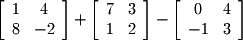Matrix/scalar addition and subtraction is analogous to vector/scalar addition and subtraction in that every entry is operated on individually. Likewise, matrix/matrix addition and subtraction is analogous to vector/vector addition and subtraction.
Excercise 3-1.
Compute the following: